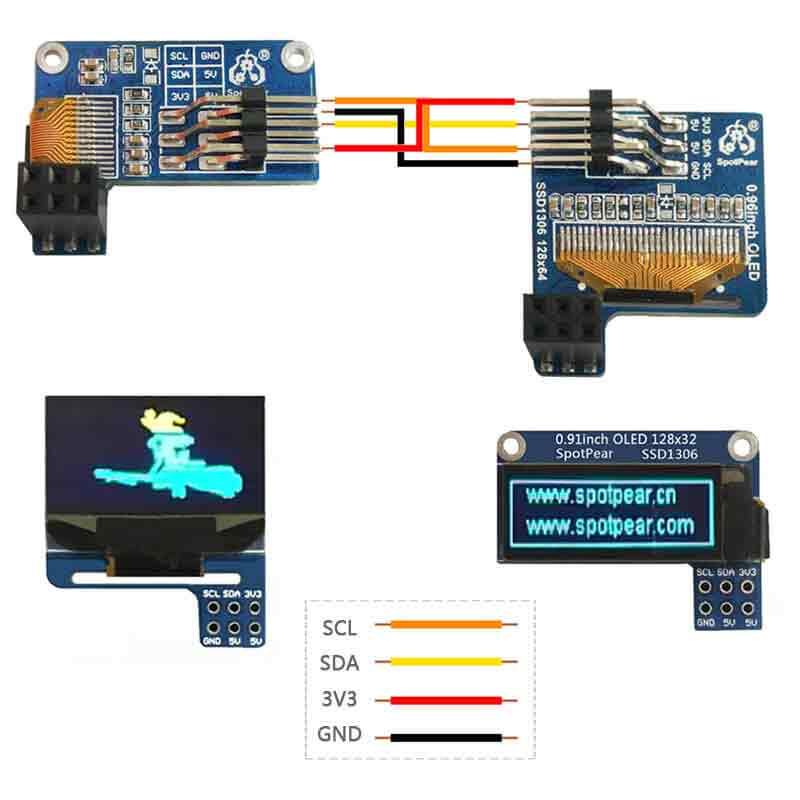•• (0)
• Account
• sales

+86-0755-88291180

# Raspberry Pi dual oled screen display User Guide (0.96+0.91 SSD1306)

## 【Preparatory work】

Before you begin, you need the following items:

[] Raspberry Pi

[] 0.91 128x32 SSD1306 OLED + 0.96 128x64 SSD1306 OLED

[] Python

Hardware connectionRun I2C interface

Restart the Raspberry Pi after ensuring that the I2C interface is turned on.

You can use the following command to find the address of the I2C device connected to the raspberry pi:

sudo i2cdetect -y 1

The running result is as shown in the figure (there are two addresses here, 0x3C is the default physical address of the oled module, and 0x3D is the modified address of the oled).

Install luma.oled library

Next, you need to install the luma.oled library on the raspberry pi. You can install in the terminal by the following command:

sudo pip3 install luma.oled

Test Python program

Before running the code, you need to create a new python file named SSD 1306 _ OLED _ 128x64 _ bad _ apple.py in Raspberry Pie (the file name can be customized).

sudo nano double_ssd1306oled.py

Combine the above steps and drive the SSD1306 OLED screen using the luma.oled library. The following is a complete Python program for driving SSD1306 OLED screen on raspberry pi:

import os

import time

from PIL import Image, ImageSequence

from PIL import ImageFont, ImageDraw

from luma.core.interface.serial import i2c

from luma.core.render import canvas

from luma.oled.device import ssd1306

device_64 = ssd1306(serial_64, width=128, height=64)

device_32 = ssd1306(serial_32, width=128, height=32)

# Return CPU temperature as a character string

def getCPUtemperature():

return(res.replace("temp=","").replace("'C\n",""))

# Return RAM information (unit=kb) in a list

# Index 0: total RAM

# Index 1: used RAM

# Index 2: free RAM

def getRAMinfo():

p = os.popen('free')

i = 0

while 1:

i = i + 1

if i==2:

return(line.split()[1:4])

# Return % of CPU used by user as a character string

def getCPUuse():

return(str(os.popen("top -n1 | awk '/Cpu\(s\):/ {print \$2}'").readline().strip()))

# Return information about disk space as a list (unit included)

# Index 0: total disk space

# Index 1: used disk space

# Index 2: remaining disk space

# Index 3: percentage of disk used

def getDiskSpace():

p = os.popen("df -h /")

i = 0

while 1:

i = i +1

if i==2:

return(line.split()[1:5])

def draw_text(text, width, height, fontsize):

font = ImageFont.truetype("arial.ttf", fontsize)

with canvas(device_32) as draw:

draw.rectangle(device_32.bounding_box, outline="white", fill="black")

# Calculate the width of the text using textlength() instead of textsize()

w = font.getlength(text)

# Calculate the height of the text by subtracting the font descent from the font ascent

ascent, descent = font.getmetrics()

h = ascent - descent

# Calculate the position of the text

x = (width - w) // 2

y = (height - h) // 2

# Draw the text using the new width and height values

draw.text((10, 1), text, font=font, fill="white")

def image_gif():

# Open a GIF file and create an Image object for each frame

frames = []

for frame in ImageSequence.Iterator(img):

frames.append(frame.convert("1").resize((128,64)))

while True:

# Display each image with an idea

for frame in frames:

device_64.display(frame)

time.sleep(0.01)

# def computer_resources(flag):

#     while not flag.is_set():

#         # CPU informatiom

#         CPU_use = getCPUuse()

#         CPU_tmp = getCPUtemperature()

#         # RAM information

#         # Output is in kb, here I convert it in Mb for readability

#         RAM_stats = getRAMinfo()

#         RAM_total = round(int(RAM_stats) / 1000,1)

#         RAM_used = round(int(RAM_stats) / 1000,1)

#         RAM_free = round(int(RAM_stats) / 1000,1)

#         # Disk information

#         DISK_stats = getDiskSpace()

#         DISK_total = DISK_stats

#         DISK_used = DISK_stats

#         DISK_perc = DISK_stats

#         #

#         status_text = "CPU_use:  "+CPU_use+"\nCPU_tmp:  "+CPU_tmp

#         draw_text(status_text, device_32.width, device_32.height, 12)

def computer_resources():

# Show some text and wait for 2 seconds

draw_text("www.spotpear.cn\nhttp://www.spotpear.com\n", device_32.width, device_32.height, 12)

time.sleep(2)

while True:

CPU_use = getCPUuse()

CPU_tmp = getCPUtemperature()

# RAM information

# Output is in kb, here I convert it in Mb for readability

RAM_stats = getRAMinfo()

RAM_total = round(int(RAM_stats) / 1000,1)

RAM_used = round(int(RAM_stats) / 1000,1)

RAM_free = round(int(RAM_stats) / 1000,1)

# Disk information

DISK_stats = getDiskSpace()

DISK_total = DISK_stats

DISK_used = DISK_stats

DISK_perc = DISK_stats

#

status_text = "CPU_use:  "+CPU_use+"\nCPU_tmp:  "+CPU_tmp

draw_text(status_text, device_32.width, device_32.height, 12)

if __name__ == '__main__':

t1.start()

t2.start()

t1.join()

t2.join()

This program realizes the display effect of gif, so it must have a corresponding gif file, otherwise the program can't run normally.

How to convert a video into a gif refer to this tutorial: Converting MP4 video into gif

Program execution effect

Input the above code into python file, save and exit, and input the following code in Raspberry Pi terminal to execute the program:

sudo python3 double_ssd1306oled.py

Price： \$2.5/2.9
Part Number： Pi OLED Module
Brand： Spotpear
SKU： 0105226